Next: Some General Remarks Up: Introduction Previous: Remembrance of Things Past   Index

# The Goal of the Course

In this course we will reorganize all of the number facts with which you are familiar. We will make a small number of assumptions or axioms about numbers, (thirteen assumptions in all, in definitions (2.48), (2.100), and (5.21)). The first twelve assumptions will be familiar number facts. The last assumption may not look familiar, but I hope it will seem as plausible as things you have assumed about numbers in the past. You will not be permitted to assume any facts about numbers other than the thirteen stated assumptions. For example, we will not assume that, or that, so you will not be allowed to assume this. (These facts will be proved in theorems 2.66 and 2.84.) You will not be allowed to assume that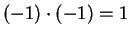, or that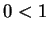. (These facts will follow from exercise 2.77c and corollary 2.104.) We will not justify the representation of numbers by points on a line, so no proofs can depend on pictures of graphs of functions. On the basis of our assumptions about real numbers, we will construct a more general class of complex numbers, in which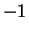, and in fact every number, has a square root. Many results about the algebra and calculus of real functions will be shown to hold for complex functions.

Occasionally I will draw pictures to motivate proofs, but the proofs themselves will not depend on the pictures. The goal of the course is to contemplate the very nature of numbers by thought alone, practising it not for the purpose of buying and selling like merchants and hucksters, butto make easier the change from the world of becoming to real being and truth.''

Sometimes in examples or remarks I will use arguments depending on similar triangles or trigonometric identities, but my theorems and definitions will depend only on my assumptions. I will also refer to integers and rational numbers in examples before I give the formal definitions, but no theorems will involve integers until they have been defined. Nothing in this course will be trivial or obvious or clear. If you come across these words, it probably means that I am engaging in a mild deception. Beware.

In theorem 2.66 we will prove the well known fact that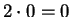. In (12.57), we will prove the less well known fact that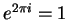. The fact that we can derive the last not-so-obvious result from our thirteen assumptions is somewhat remarkable.Next: Some General Remarks Up: Introduction Previous: Remembrance of Things Past   Index